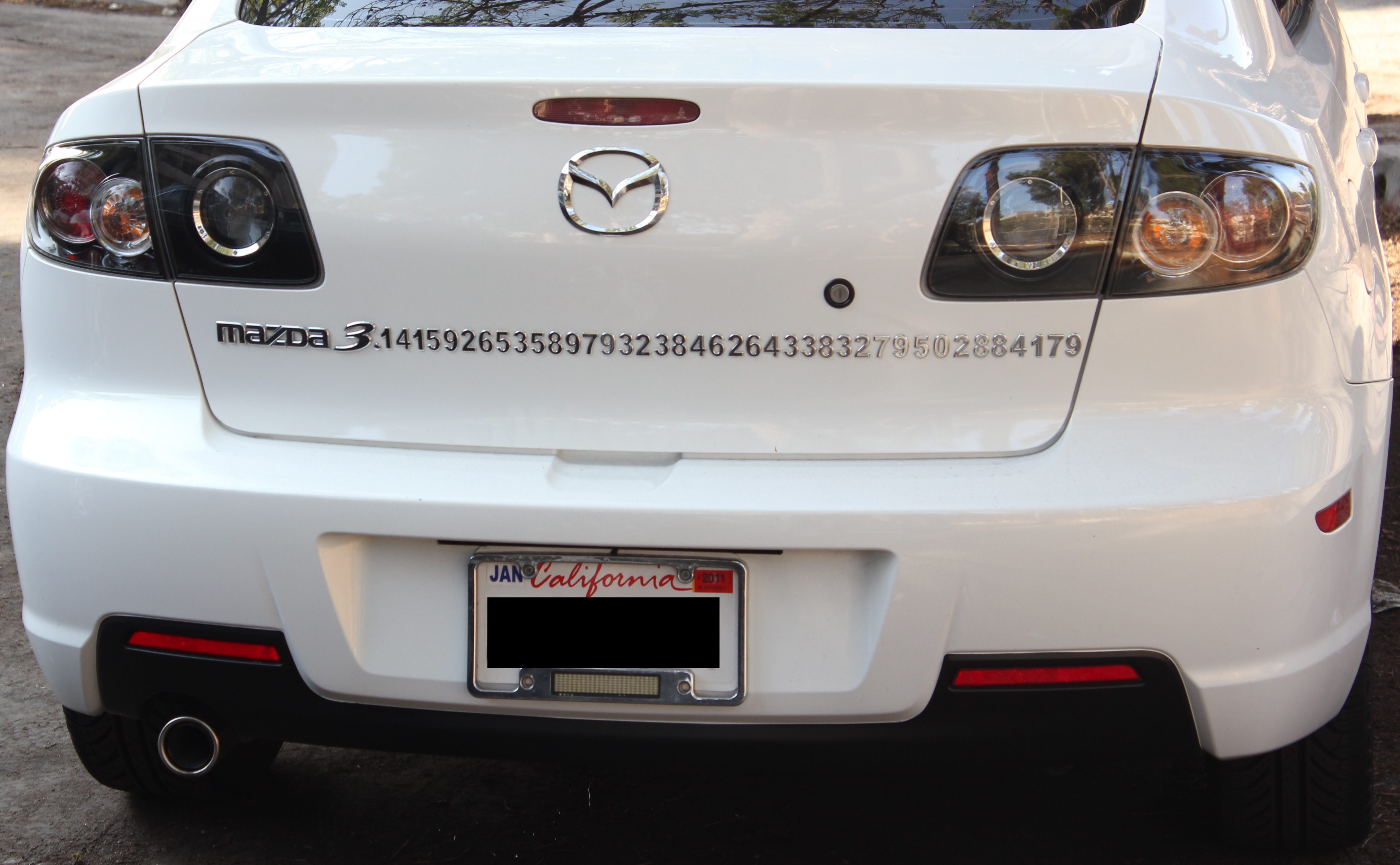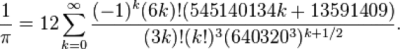Blogs

# Making Pi

### How a computer program calculated 13 trillion digits of pi

###### Ryan F. Mandelbaum• March 14, 2016##### This person really likes pi. [Image Credit: Basil D Soufi | CC BY-SA 3.0]

If we had an infinitely powerful computer, an infinite amount of time and an infinite amount of memory, we could calculate all of the digits of pi.

Infinity is pretty big. And by that I mean any attempt to visualize infinity is silly because infinity doesn’t have any boundaries you can visualize it in. Say you had a camera that could take a picture of something infinitely large and you wanted to zoom out so you could fit everything on the screen. You could zoom out for your whole life, then pass the task onto your children and so on and so forth until the universe ends, and you wouldn’t have a fraction of the total image. Yet somehow, computer whiz Alex Yee was able to write a program that could (if there was infinite memory in the world) calculate all the digits of pi.

That’s because in math, infinity is a journey, not a destination.

Most recently, someone with the username houkouonchi used Yee’s program to print a new world record: 13 trillion digits of pi. While Yee’s program is simple, houkouonchi needed to supply the huge amount of computing power to calculate and save all of those digits. While we simply don’t need that many digits of pi for any calculation, Yee’s program has an application outside of calculating all the digits of pi; it can also serve as a test for how well a computer can run. Since Yee’s program involves running a simple math problem very quickly for a long period of time without crashing, it tests how well and quickly the computer can do calculations.

To actually calculate all of the digits of pi, Yee’s program uses something called a series. Series are the result of taking an infinite list of numbers and adding them all together. Most commonly, series have some rule that ties each member of the list together. For example, 1+2+3+4 … is a series. So is -1+1-1+1 …, or 1/1+1/2+1/3 … or any sum of numbers. In the first example, I just added all of the numbers. In example 2, I multiplied -1 by itself for each number that exists: (-1)+(-1*-1)+(-1*-1*-1). In the third example, I took 1 and divided it by its position in the list.

There are two different behaviors that series can have as you add more and more list items. Either the sum can approach some destination or the sum can just get bigger and bigger on its way to infinity. 1+1+1+1 … will just go to infinity, but 1/2+1/4+1/8 … (that’s the sum of one divided by every power of 2) will eventually kiss 1 as the numbers you’re adding to your subtotal get smaller and smaller. It’s the difference between stacking papers on top of each other forever and using an infinite number of smaller and smaller scraps to make a single sheet of paper. They’re both infinite sums, but one will eventually fill the whole universe with paper while the other can sit on your desk.

In the latter example, you basically have an infinite number of paper pieces and when you add them all together they approximate the number 1. The first piece is half a sheet, then a quarter, then an eighth and so on. We don’t need to use the series of paper pieces to figure out the value of one because we already know what it is: one. We don’t know exactly how big pi is, but we can keep getting closer to it by using an infinite series, just like we did for the number one. It’s called the Chudnovsky algorithm, and it looks like this:Image via Wikipedia

See? Easy.

It looks complicated, but all it says is: “If you want to know what one divided by pi is, replace the letter k to the right of the ∑ sign with every number from zero to infinity. Then, add those values together one at a time, and multiply the whole thing by twelve.” The more numbers you add, the more digits of pi you’ll get.

The Chudnovsky algorithm is one of many series that you can use to get pi. However, Yee used it because it gets you the most digits the fastest – every time you add another term in the series, you get an average of 14 more digits of pi.

That’s all Yee’s program does; it’s a very powerful calculator that he started working on in high school. The pi-maker, houkouonchi, simply plugged in the Chudnovsky algorithm and started the program. And in October 2014, after a year straight running the program and dealing with hardware issue, houkouonchi’s computer spit out 13 trillion digits of pi.

Of course, 13 trillion isn’t even a dent in the total number of digits of pi, because there are infinity decimal places. But programs like Yee’s help us push the limit of our computers and our minds and show just how vast pi really is.

#### Share#### Ryan F. Mandelbaum

makes his triumphant return to New York after spending two years battling flight delays, polar vortices and indigestion while implementing healthcare software for Epic Systems in Madison, Wisconsin. Ryan earned his B.A. in physics and mathematics at Columbia University and studied heavy ion collisions at CERN during the summer of 2012 (spot him in Particle Fever wearing his CERN basketball jersey). Ryan will be the first Billboard Hot 100 artist to rap about supersymmetry.

Subscribe